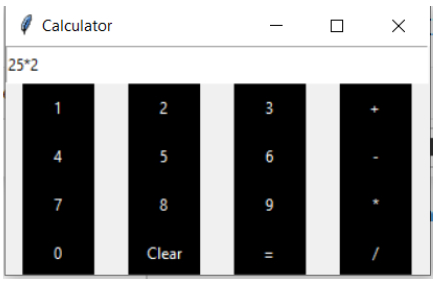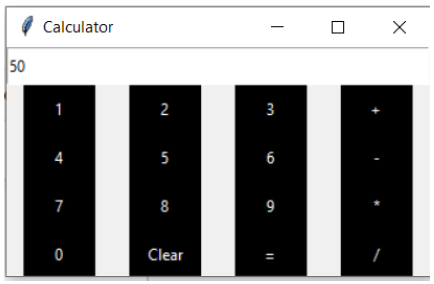# Simple GUI calculator using Tkinter in Python

In this tutorial, we are going to create a simple GUI calculator using the Tkinter module. Tkinter is builtin the Python module for developing the GUI application. It's easy to use and comes with Python. We can visualize our data with GUI applications.

Let's see how to create a simple GUI calculator.

• Import everything from the Tkinter using *.

• Create an interface for the calculator.

• Create an input function that enters a number into the input field.

• Create an apparent function that wipes everything from the input field.

• And finally, evaluate function that computes and gives the result of the expression.

## Example

# importing everyting from tkinter
from tkinter import *
# expression to access among all the functions
expression = ""
# functions
def input_number(number, equation):
# accessing the global expression variable
global expression
# concatenation of string
expression = expression + str(number)
equation.set(expression)
def clear_input_field(equation):
global expression
expression = ""
# setting empty string in the input field
equation.set("Enter the expression")
def evaluate(equation):
global expression
# trying to evaluate the expression
try:
result = str(eval(expression))
# showing the result in the input field
equation.set(result)
# setting expression to empty string
expression = ""
except:
# some error occured
# showing it to the user equation.set("Enter a valid expression")
expression = ""
# creating the GUI
def main():
# main window window = Tk()
# setting the title of GUI window
window.title("Calculator")
# set the configuration of GUI window
window.geometry("325x175")
# varible class instantiation
equation = StringVar()
# input field for the expression
input_field = Entry(window, textvariable=equation)
input_field.place(height=100)
# we are using grid position
# for the arrangement of the widgets
# settin the placeholder message for users
equation.set("Enter the expression")
# creating buttons and placing them at respective positions
_1 = Button(window, text='1', fg='white', bg='black', bd=0, command=lambda: input_number(1, equation), height=2, width=7)
_1.grid(row=2, column=0)
_2 = Button(window, text='2', fg='white', bg='black', bd=0, command=lambda: input_number(2, equation), height=2, width=7)
_2.grid(row=2, column=1)
_3 = Button(window, text='3', fg='white', bg='black', bd=0, command=lambda: input_number(3, equation), height=2, width=7)
_3.grid(row=2, column=2)
_4 = Button(window, text='4', fg='white', bg='black', bd=0, command=lambda: input_number(4, equation), height=2, width=7)
_4.grid(row=3, column=0)
_5 = Button(window, text='5', fg='white', bg='black', bd=0, command=lambda: input_number(5, equation), height=2, width=7)
_5.grid(row=3, column=1)
_6 = Button(window, text='6', fg='white', bg='black', bd=0, command=lambda: input_number(6, equation), height=2, width=7)
_6.grid(row=3, column=2)
_7 = Button(window, text='7', fg='white', bg='black', bd=0, command=lambda: input_number(7, equation), height=2, width=7)
_7.grid(row=4, column=0)
_8 = Button(window, text='8', fg='white', bg='black', bd=0, command=lambda: input_number(8, equation), height=2, width=7)
_8.grid(row=4, column=1)
_9 = Button(window, text='9', fg='white', bg='black', bd=0, command=lambda: input_number(9, equation), height=2, width=7)
_9.grid(row=4, column=2)
_0 = Button(window, text='0', fg='white', bg='black', bd=0, command=lambda: input_number(0, equation), height=2, width=7)
_0.grid(row=5, column=0)
plus = Button(window, text='+', fg='white', bg='black', bd=0, command=lambda: input_number('+', equation), height=2, width=7)
plus.grid(row=2, column=3)
minus = Button(window, text='-', fg='white', bg='black', bd=0, command=lambda: input_number('-', equation), height=2, width=7)
minus.grid(row=3, column=3)
multiply = Button(window, text='*', fg='white', bg='black', bd=0, command=lambda:  input_number('*', equation), height=2, width=7)
multiply.grid(row=4, column=3)
divide = Button(window, text='/', fg='white', bg='black', bd=0, command=lambda: input_number('/', equation), height=2, width=7)
divide.grid(row=5, column=3)
equal = Button(window, text='=', fg='white', bg='black', bd=0, command=lambda: evaluate(equation), height=2, width=7)
equal.grid(row=5, column=2)
clear = Button(window, text='Clear', fg='white', bg='black', bd=0, command=lambda: clear_input_field(equation), height=2, width=7)
clear.grid(row=5, column=1)
# showing the GUI
window.mainloop()
# start of the program
if __name__ == '__main__':
main()



## Output

If you run the above program, you will get a simple calculator as follows.Result of the above expression generated after pression = button.## Conclusion

If you have any doubts in the tutorial, mention them in the comment section.